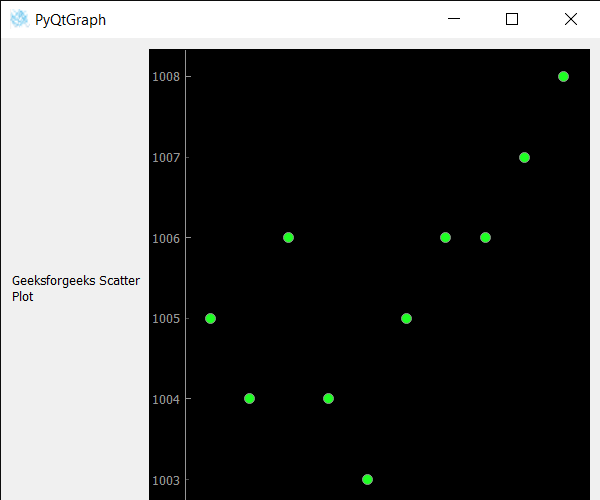Related Articles

# PyQtGraph – Moving Data of Scatter Plot Graph

• Last Updated : 24 Oct, 2020

In this article we will see how we can move the data of scatter plot graph in the PyQtGraph module. PyQtGraph is a graphics and user interface library for Python that provides functionality commonly required in designing and science applications. Its primary goals are to provide fast, interactive graphics for displaying data (plots, video, etc.). A scatter plot (aka scatter chart, scatter graph) uses dots to represent values for two different numeric variables. It is a type of plot or mathematical diagram using Cartesian coordinates to display values for typically two variables for a set of data. The position of each dot on the horizontal and vertical axis indicates values for an individual data point. Moving data means that all the spots position get moved.
We can create a plot window and create scatter plot graph on it with the help of commands given below.

```# creating a pyqtgraph plot window
plt = pg.plot()

# creating a scatter plot graph of size = 10
scatter = pg.ScatterPlotItem(size=10)
```

In order to do this we use moveBy method with the scatter plot graph object
Syntax : scatter.moveBy(x, y)
Argument : It takes two integers as argument
Return : It returns None

Below is the implementation

## Python3

 `# importing Qt widgets``from` `PyQt5.QtWidgets ``import` `*`` ` `# importing system``import` `sys`` ` `# importing numpy as np``import` `numpy as np`` ` `# importing pyqtgraph as pg``import` `pyqtgraph as pg``from` `PyQt5.QtGui ``import` `*``from` `PyQt5.QtCore ``import` `*`` ` `class` `Window(QMainWindow):`` ` `    ``def` `__init__(``self``):``        ``super``().__init__()`` ` `        ``# setting title``        ``self``.setWindowTitle(``"PyQtGraph"``)`` ` `        ``# setting geometry``        ``self``.setGeometry(``100``, ``100``, ``600``, ``500``)`` ` `        ``# icon``        ``icon ``=` `QIcon(``"skin.png"``)`` ` `        ``# setting icon to the window``        ``self``.setWindowIcon(icon)`` ` `        ``# calling method``        ``self``.UiComponents()`` ` `        ``# showing all the widgets``        ``self``.show()`` ` `    ``# method for components``    ``def` `UiComponents(``self``):`` ` `        ``# creating a widget object``        ``widget ``=` `QWidget()`` ` `        ``# creating a label``        ``label ``=` `QLabel(``"Geeksforgeeks Scatter Plot"``)`` ` `        ``# making label do word wrap``        ``label.setWordWrap(``True``)`` ` `        ``# creating a plot window``        ``plot ``=` `pg.plot()`` ` `        ``# number of points``        ``n ``=` `300`` ` `        ``# creating a scatter plot item``        ``# of size = 10``        ``# using brush to enlarge the of green color``        ``scatter ``=` `pg.ScatterPlotItem(``            ``size``=``10``, brush``=``pg.mkBrush(``30``, ``255``, ``35``, ``255``))`` ` `        ``# data for x-axis``        ``x_data ``=` `[``1``, ``2``, ``3``, ``4``, ``5``, ``6``, ``7``, ``8``, ``9``, ``10``]`` ` `        ``# data for y-axis``        ``y_data ``=` `[``5``, ``4``, ``6``, ``4``, ``3``, ``5``, ``6``, ``6``, ``7``, ``8``]`` ` `        ``# setting data to the scatter plot``        ``scatter.setData(x_data, y_data)`` ` `        ``# add item to plot window``        ``# adding scatter plot item to the plot window``        ``plot.addItem(scatter)`` ` `        ``# Creating a grid layout``        ``layout ``=` `QGridLayout()`` ` `        ``# minimum width value of the label``        ``label.setMinimumWidth(``130``)`` ` `        ``# setting this layout to the widget``        ``widget.setLayout(layout)`` ` `        ``# adding label in the layout``        ``layout.addWidget(label, ``1``, ``0``)`` ` `        ``# plot window goes on right side, spanning 3 rows``        ``layout.addWidget(plot, ``0``, ``1``, ``3``, ``1``)`` ` `        ``# setting this widget as central widget of the main widow``        ``self``.setCentralWidget(widget)`` ` `        ``# moving the data of scatter plot``        ``scatter.moveBy(``1000``, ``1000``)`` ` `# create pyqt5 app``App ``=` `QApplication(sys.argv)`` ` `# create the instance of our Window``window ``=` `Window()`` ` `# start the app``sys.exit(App.``exec``())`

Output :Attention geek! Strengthen your foundations with the Python Programming Foundation Course and learn the basics.

To begin with, your interview preparations Enhance your Data Structures concepts with the Python DS Course. And to begin with your Machine Learning Journey, join the Machine Learning – Basic Level Course

My Personal Notes arrow_drop_up